2020 NBA Rookie of the Year Odds
-177
0.56 to 1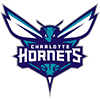LaMelo Ball
46.0% implied probability

+275
2.75 to 1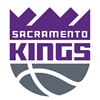Tyrese Haliburton
19.2% implied probability

+800
8 to 1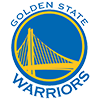James Wiseman
8.0% implied probability

+1600
16 to 1Anthony Edwards
4.2% implied probability

+2000
20 to 1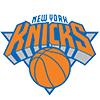Immanuel Quickley
3.4% implied probability

+3300
33 to 1Cole Anthony
2.1% implied probability

+5000
50 to 1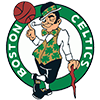Payton Pritchard
1.4% implied probability

+5000
50 to 1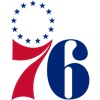Tyrese Maxey
1.4% implied probability

+5000
50 to 1Xavier Tillman
1.4% implied probability

No changes have been recorded yet.
+5000
50 to 1Obi Toppin
1.4% implied probability

+8000
80 to 1Isaac Okoro
0.9% implied probability

+8000
80 to 1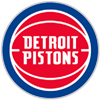Saddiq Bey
0.9% implied probability

+8000
80 to 1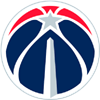Deni Avdija
0.9% implied probability

+10000
100 to 1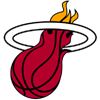Precious Achiuwa
0.7% implied probability

+10000
100 to 1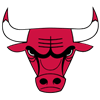Patrick Williams
0.7% implied probability

+10000
100 to 1Desmond Bane
0.7% implied probability

+15000
150 to 1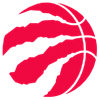Malachi Flynn
0.5% implied probability

+15000
150 to 1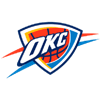Aleksej Pokusevski
0.5% implied probability

+15000
150 to 1Facundo Campazzo
0.5% implied probability

No changes have been recorded yet.
+15000
150 to 1Bol Bol
0.5% implied probability

+15000
150 to 1Killian Hayes
0.5% implied probability

+15000
150 to 1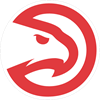Onyeka Okongwu
0.5% implied probability

+15000
150 to 1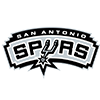Devin Vassell
0.5% implied probability

+15000
150 to 1Theo Maledon
0.5% implied probability

+25000
250 to 1Tyrell Terry
0.3% implied probability

+25000
250 to 1Jalen Smith
0.3% implied probability

+25000
250 to 1Isaiah Stewart
0.3% implied probability

+25000
250 to 1Josh Green
0.3% implied probability

+25000
250 to 1Jaden McDaniels
0.3% implied probability

+25000
250 to 1Aaron Nesmith
0.3% implied probability

+25000
250 to 1Leandro Bolmaro
0.3% implied probability

+25000
250 to 1Tre Jones
0.3% implied probability

+25000
250 to 1R.J. Hampton
0.3% implied probability

+25000
250 to 1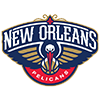Kira Lewis
0.3% implied probability